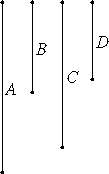# Proposition 12

If any number of numbers are proportional, then one of the antecedents is to one of the consequents as the sum of the antecedents is to the sum of the consequents.Let A, B, C, and D be as many numbers as we please in proportion, so that A is to B as C is to D.

I say that A is to B as the sum of A and C is to the sum of B and D.

Since A is to B as C is to D, therefore A is the same part or parts of B as C is of D. Therefore the sum of A and C is the same part or parts of the sum of B and D that A is of B.

VII.Def.20

Therefore A is to B as the sum of A and C is to the sum of B and D.

Therefore, if any number of numbers are proportional, then one of the antecedents is to one of the consequents as the sum of the antecedents is to the sum of the consequents.

Q.E.D.

## Guide

This proposition is the numerical analogue of V.12. Symbolically,

if a1 : b1 = a2 : b2 = ... = an : bn,

then each of these ratios also equals the ratio

(a1 + a2 + ... + an) : (b1 + b2 + ... + bn).

Euclid takes n to be 2 in his proof.

This proposition is used in VII.15 , VII.20, and IX.35.